Question
1. Design and implement a 4 to 1 multiplexer circuit using CMOS transistors.​​​ (30 Marks)
(Note: Students are expected to design the circuit with truth table, solve the output expression by use of K Map or suitable circuit Reduction technique and implement using CMOS transistors.)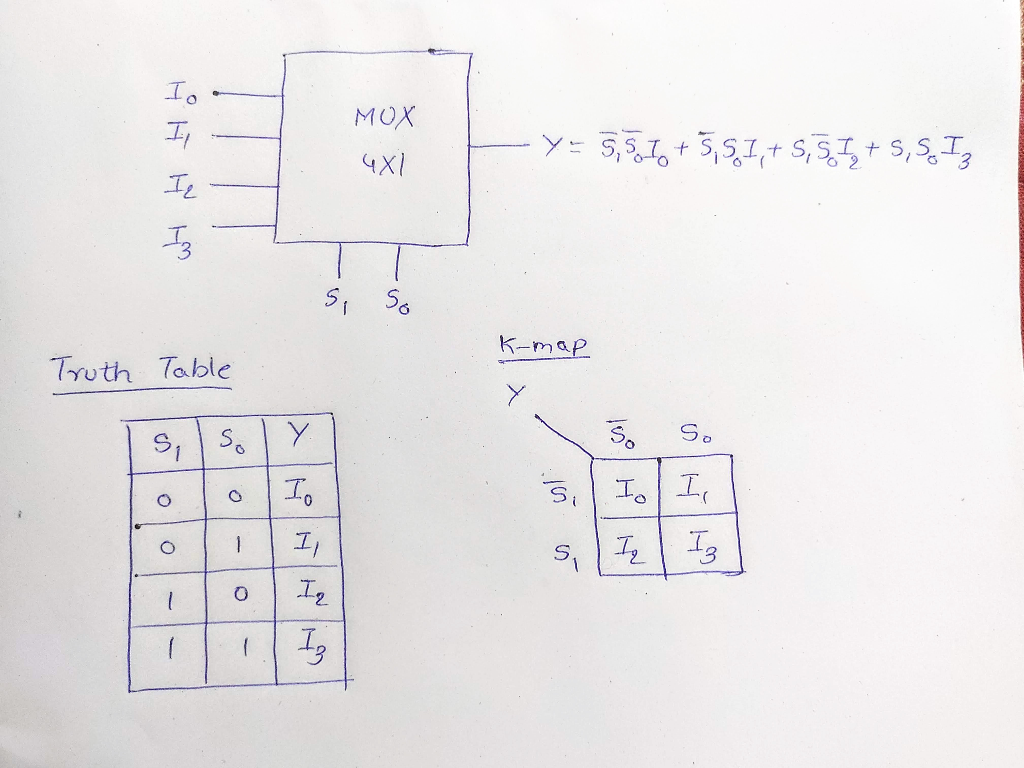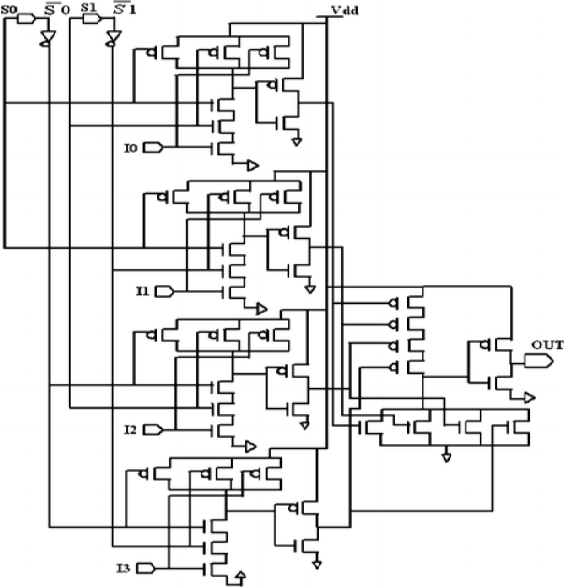#### Earn Coins

Coins can be redeemed for fabulous gifts.

Similar Homework Help Questions
• ### Questions 1. Design and implement a full subtractor circuit using CMOS transistors (30 Marks) (Note: Students are expected to design the circuit with truth table, solve the output expression (by use...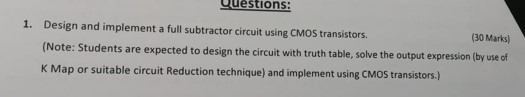Questions 1. Design and implement a full subtractor circuit using CMOS transistors (30 Marks) (Note: Students are expected to design the circuit with truth table, solve the output expression (by use of Map or suitable circuit Reduction technique) and implement using CMOS transistors.) Questions 1. Design and implement a full subtractor circuit using CMOS transistors (30 Marks) (Note: Students are expected to design the circuit with truth table, solve the output expression (by use of Map or suitable circuit Reduction...

• ### 1. Design and implement a full subtractor circuit using CMOS transistors. (30 Marks) (Note: Students are expected to des...

1. Design and implement a full subtractor circuit using CMOS transistors. (30 Marks) (Note: Students are expected to design the circuit with truth table, solve the output expression (by use of K Map or suitable circuit Reduction technique) and implement using CMOS transistors.) p.s: simplify the k map equation (with minimum expressions) then draw the cmos transistors.

• ### Design and implement a 4 bit- binary to gray code converter using CMOS transistors.                             ...

Design and implement a 4 bit- binary to gray code converter using CMOS transistors.                                (30 Marks) (Note: Students are expected to design the circuit with truth table, solve the output expression (by use of K Map or suitable circuit Reduction technique) and implement using CMOS transistors.)

• ### Design and implement a 4 bit- gray to binary code converter using CMOS transistors.               (Note: Students are expected to design the circuit with truth table, solve the output expression (by...

Design and implement a 4 bit- gray to binary code converter using CMOS transistors.               (Note: Students are expected to design the circuit with truth table, solve the output expression (by use of K Map or suitable circuit Reduction technique) and implement using CMOS transistors.)

• ### Design and implement a combinational circuit with four inputs w,x, y and z and four outputs A, B ,C and D using CMOS transistors. When the binary input is 0, 1, 2,3,4,5,6 or 7 the binary output is fi...

Design and implement a combinational circuit with four inputs w,x, y and z and four outputs A, B ,C and D using CMOS transistors. When the binary input is 0, 1, 2,3,4,5,6 or 7 the binary output is five greater than the input. When the binary input is 8,,10,11,12,13,14 or 15 the binary output is seven less than the input. for question (a) find the troth table for the inputs (ABCD) then implement using K-map to find the equations to...

• ### Design and implement the following circuit with four inputs and four outputs using CMOS transistors. The first output...

Design and implement the following circuit with four inputs and four outputs using CMOS transistors. The first output is high when the binary value of the input is less than or equal to7 Draw the mask layout with Ln = Lp= 0.6 μm, Wn= 4.8 μm and Wp= 8.4 μm using 0.6 μm technology. Also simulate the design using microwind tool and verify the outputs.

• ### 1. a. Design and implement a 2 bit comparator circuit using CMOS transistors(Greater than,less than, equal...

1. a. Design and implement a 2 bit comparator circuit using CMOS transistors(Greater than,less than, equal to or reverse). b. Draw the mask layout with Ln = Lp= 0.6 μm, Wn= 4.8 μm and Wp= 7.8 μm using 0.6 μm technology. Also simulate the design using microwind tool and verify the outputs.

• ### 1. a. Design and implement a 2 bit comparator circuit using CMOS transistors(Greater than,less than, equal to or re...

1. a. Design and implement a 2 bit comparator circuit using CMOS transistors(Greater than,less than, equal to or reverse). b. Draw the mask layout with Ln = Lp= 0.6 μm, Wn= 4.8 μm and Wp= 7.8 μm using 0.6 μm technology. Also simulate the design using microwind tool and verify the outputs.

• ### Logic Q5:(7: Marks) Use the circuit below to answer the following questions: a. Implement this design...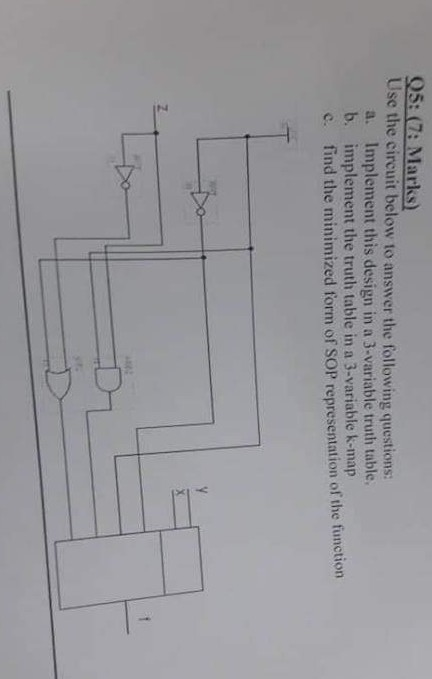Logic Q5:(7: Marks) Use the circuit below to answer the following questions: a. Implement this design in a 3-variable truth table, b. implement the truth table in a 3-variable k-map c. find the minimized form of SOP representation of the funct iorn

• ### 3. Design of a 2 input XNOR gate using CMOS transistors, a. Realize the 2 input...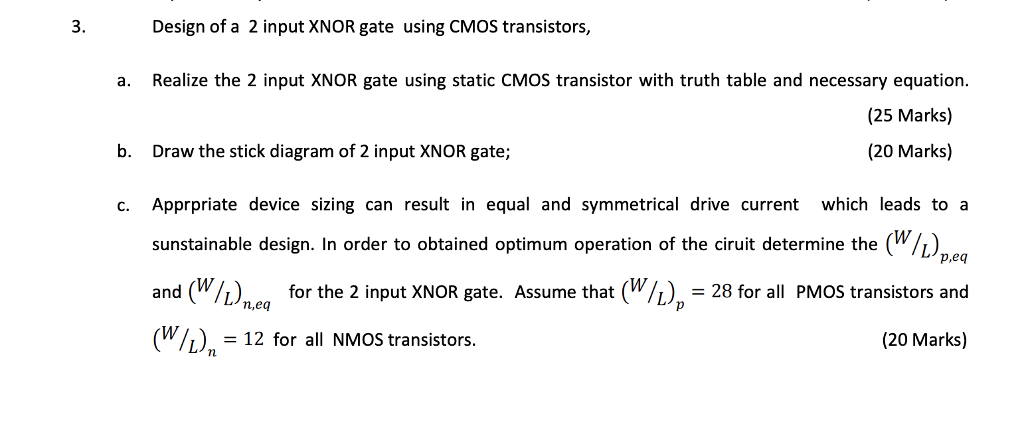3. Design of a 2 input XNOR gate using CMOS transistors, a. Realize the 2 input XNOR gate using static CMOS transistor with truth table and necessary equation. (25 Marks) (20 Marks) b. Draw the stick diagram of 2 input XNOR gate; c.Apprpriate device sizing can result in equal and symmetrical drive current which leads to a sunstainable design. In order to obtained optimum operation of the cirut determine the(Whpe and (W/L) for the 2 input XNOR gate. Assume that...